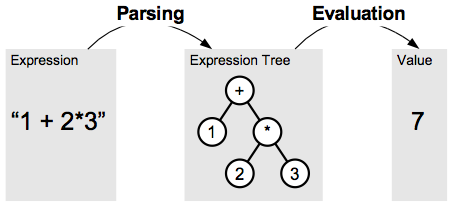# Introduction

## Overview

Jep.Net is a .NET library for parsing and evaluating mathematical expressions. With this package you can take formulas as strings, and instantly evaluate them. Many common mathematical functions and constants are built-in and ready to use. But in addition, you can extend Jep.Net with your own user defined variables, constants, and functions.

### Features

• Small size (only 154KB as dll)
• Fast evaluation
• Support for arbitrary-precision arithmetic
• Includes common math functions and predefined constants
• Extendable through user defined functions
• Supports Boolean expressions (!, &&, ||, <, >, !=, ==, >=, and <=)
• Support for strings, arrays, and complex numbers
• Support for implicit multiplication (allows use of expressions such as "3x" instead of "3*x")
• Support for assignment expressions (x = 5)
• Allows choice between declared and undeclared variables
• Supports Unicode characters (including Greek symbols)
• Extensive documentation

## Parsing and Evaluation

Evaluating a string expression with Jep.Net is a two step process as shown in the figure below. First the expression is parsed, bringing it from a string representation into a tree representation. The tree representation is a structured representation of the expression which allows a simple and fast evaluation of the expression in a second step. The following sections will discuss these two steps in more detail.### Parsing

One can describe parsing in general terms as taking a series of characters as input and producing a structured representation of the information contained in that set of characters. The focus here is on mathematical expressions although parsing is also used for other areas such as natural language parsing for example.

Math expressions can be represented by a tree data structure where numbers, variables, operators and functions all are represented by nodes. Numbers and variables are represented by so-called child nodes, while operators and functions are represented by parent nodes. The parent nodes operate on their child nodes. For example, the expression "1+2*3" can be represented by a tree with five nodes, as shown above.

You might ask why the multiplication node is a child of the plus node. The reason for this is operator precedence. Operators have an order of precedence which needs to be obeyed when parsing expressions. The precedence of multiplication is higher than that of addition. So, for the above example, one needs to first multiply 2 and 3 before adding the result to 1. In the tree, this is represented by grouping the the numbers 2 and 3 as children of the multiplication operator. Finally, the multiplication node itself is a child of the addition node, expressing that the result of the multiplication is used for the addition operation. The parser is responsible for following the precedence order as specified in the grammar.

Parsers are typically generated using parser generators such as ANTLR, SableCC, Yacc, or Bison. Jep.Net uses a custom, configurable parser that allows users to specify their own tokens and token matchers. See Configurable Parser for more info.

### Evaluation

Evaluation takes the expression from a tree representation to the value of the expression. Since the tree is built according to the operator precedence, the evaluation method does not need to know about operator precedence. The tree can be traversed using a simple method to determine the value of the expression.

A simple approach is to start from the root node (the topmost node) and apply a recursive method. This can be implemented using the visitor design pattern. A different method can be created for each node type. To evaluate an operator or function node, first the child nodes are evaluated. Then, using the values of the child nodes, the operator or function is applied to determine the value of the node itself. For nodes that are constants (numbers) or variables, the node evaluates to the value of the constant or variable. Using such a recursive approach, the tree is quickly traversed in a depth-first manner.